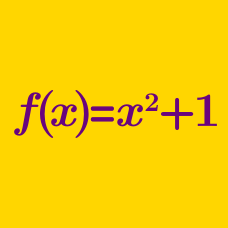Algebra

# Functions: Level 2 Challenges

Consider a function $f$ satisfying

$f\big(\sqrt{x}\big)=x^2.$

What is the value of $f(2) ?$

Suppose $f$ is a real function satisfying $f(x+f(x)) = 4f(x)$ and $f(1)=4$. What is $f(21)$?

Given that $f(2^x)+xf(2^{-x})=1$, find the value of $f(2)$.

$x * y = \frac{1}{x} + \frac{1}{y}, \quad x \# y = \frac{x+y}{x-y}$

Let the operations $\#$ and $*$ be defined as described above.

Find the value of $k$ such that

$(22 * k) \# (k * 33) = 27.$

If $f$ is a function such that $f(f(x)) = x^2 - 1$, what is the value of $f(f(f(f(3))))$?

×